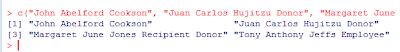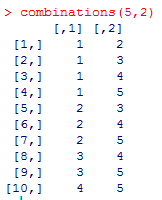## Friday, July 29, 2011

### Splitting Vectors of Uneven Strings

Suppose you have a vector of names such that the first three words in the vector contain relevant information, but there is a bunch of extraneous stuff. For example,Our goal is to collapse the first three words into one contiguous string (without the spaces) and we want to discard the extra words (because they're extraneous infromation). For a situation like this, my first thought is to use the strsplit() command, which splits vectors of strings by any specified character pattern you wish. For example, suppose that an object named myvec contains the vector of strings we would like to split into separate words. Then, the command

strsplit(myvec, " ")

produces a list of vectors of words. This is a little cumbersome because the vectors have a different length for each element in the list. Fortunately, all we want in this application is the first three words concatenated together, so some trimming will still give us the right answer. Even better, if you don't mind seeing an error message on account of trying to bind vectors of uneven lengths, we can rbind the list together using do.call() as follows:

do.call(rbind, strsplit(myvec, " "))

This still works because R recycles. For our example, we then obtain a fabulous 4x5 matrix of names:Store this matrix in the object namemat, then reduce this matrix to containing only the first three words by selecting those columns:

namemat = namemat[, 1:3]

Think of each row of this resulting matrix as a vector. If we can paste this vector together into one string, we're done. Do this using the paste() command with collapse = "". To paste() simultaneously for each row, I like to use apply. The resulting command that goes from namemat to vector of cleaned-and-concatenated strings is:

apply(namemat, MARGIN = 1, FUN = function(x){ paste(x, collapse="")})

MARGIN = 1 is for rows. Putting the pieces together, the code we developed is:

namemat = do.call(rbind, strsplit(myvec, " "))
namemat = namemat[, 1:3]
apply(namemat, MARGIN = 1, FUN = function(x){ paste(x, collapse="")})

And, this should work if your list of names is 4 lines long or 40 million lines long. That said, there was a lot of heavy lifting along the way (we created a list, collapsed a list, produced an error message as a byproduct of binding the list into a matrix, pasted each row using the collapse option and pasted quickly using apply).

There's a strange way to do this same task that doesn't require the same heavy lifting (just different heavy lifting). Use write.table() and read.table(). Start by writing the unclean initial vector to a text file without row names.

write.table(myvec, "C://vec.txt", sep = "", quote = FALSE, row.names = FALSE)

Also, turn off quoting. We do this to trick R into thinking that the spaces we want to eliminate are space delimiters. Then, read in the file using read.table() and a space delimiter.

namemat = read.table("Data/Raw/crspnames.txt", skip=1, sep = " ", quote = "", fill = TRUE)

skip =1 is to avoid reading in the variable name. The fill = TRUE argument fills in the matrix so that read.table() doesn't return an error for trying to read a non-square table. Each row of the resulting matrix namemat has a full name to be collapsed, one word per column (similar to namemat from the previous method). To complete the task, we still need to paste the first three columns together for each row. For this, use the apply-the-paste technique we used in the first method.

On a final note, if we don't mind pasting the entire vector together without the spaces (including the extraneous stuff), the easy solution is to use the gsub() command to replace spaces with nothing.

gsub(" ", "", myvec)

This method will produce an answer that is close to the one that we wanted and with a simple one line of code. Depending on your objective, this may be the best way to go -- that is, if you don't care about having extra words appended to each string.

## Monday, July 18, 2011

### Avoiding Loops in R: An Example with Principal Minors

Yesterday, I found myself wanting to compute a large subset of the second order principal minors of a matrix (diagonal-preserving minors; the ones for which the rows and columns kept are the same). Don't judge me for wanting to do this, and bear with me, there is a lesson about R programming here (mostly for me, read the comments for testing).

If you don't know what a principal minor is or forgot what it was (because most of us don't spend much time with principal minors), here is a short refresher on how to get a second-order principal minor (other orders are possible and there's a lot of related theory, but let's try to focus on this one task for now).

Step 1: Deletion. Take any matrix. Delete all but two rows and all but two columns. For example, suppose the matrix is 5x5 and let's keep rows 1 and 3 and columns 2 and 4 (lots of other combinations are possible).In the back of your mind, think how easy this is in R.

Step 2: Calculation. Once we delete the irrelevant parts of the big matrix to get our 2x2 submatrix, obtain the principal minor by taking the determinant of the resulting 2x2 matrix. For our example, we compute 3x2 - 3x1 = 3.

Both steps in this calculation are easy to implement in R. For the first step, merely use R's fantastic syntax for extracting rows and columns of matrices. If the matrix is called mat, we efficiently perform the first step by:

little_mat = mat[c(1,3), c(2,4)]

That's easy enough. With little_mat in hand, the second step is even easier.

pm = det(little_mat)

The real trick to this programming problem is picking out all of principal minors I want to compute (diagonal-preserving ones that keep the same-numbered rows and columns; little_mat won't do because c(1,3) is not c(2,4)), and doing it efficiently. I also don't want to rewrite my code as my matrix changes size.

So, what do we do? A really bad An idea that will eventually compute the right answer is to write this using for() loops.

Barring a coding error, this code should eventually work, but it will be slow (or fast depending on how you write it). If you want to run through this code many times (like I did yesterday), efficiency is at a premium and you should immediately think of faster techniques -- like using the apply() function (or preallocating your storage vector, which actually saves time).

Before moving to apply(), we need a quick way to enumerate all of the ways to keep two out of N rows. If we were counting, that's N choose 2, also known as a combination. Fortunately, this step has already been implemented in the gregmisc library with a function called combinations(). Conveniently, combinations(N, 2) will return a matrix with all of the ways to select two numbers less than or equal to N as its rows. There are other options, but this is the default, which is perfect for our application.

For example, here is some output for N=5.With this way of computing the rows/ columns we want to keep, all we need to do is put our simple code for computing a second order principal minor into a function. Let's call it minor2(). Then, use apply() to apply this function to each row (MARGIN=1 is for rows) of the combinations matrix. Here's the complete set of code that will work if you have a matrix called mat for which you want the diagonal-second order principal minors.

Because we avoid doing loops, this method is much faster. For a big project, this added efficiency is worth it. More generally, this technique of using apply() to avoid repeatedly looping is a good lesson to apply to other programming problems.

Update: Thanks to Berend for going through the trouble of system.time() testing the code in this post (and spiceman for a good comment as well). Read on into the comments for more, but for a preview of what we learned: (1) The copying via c() is what slows the loop down, (2) apply is slightly slower than writing the loop yourself, (3) combn(), the combinations() alternative, is really slow, and (4) one should always stand behind system.time() methods before talking about the efficiency of a method.

I much appreciate the feedback. One of the reasons I have this blog is to learn more about R (and those comments were definitely informative).